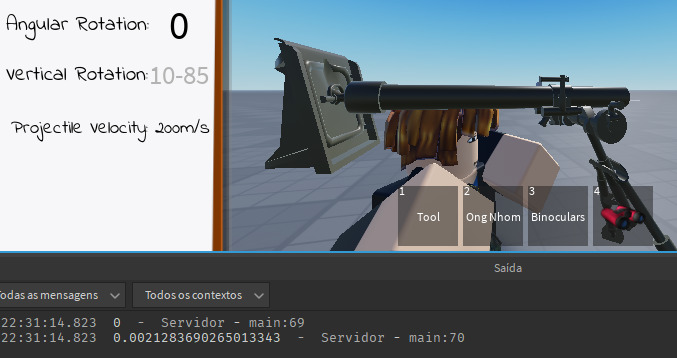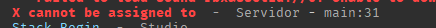# Projectile shot calculations

Well… I’m making a mortar, and I’m wanting to make a system that calculates the explosion location of the projectile launched. And I also wanted to know how to calculate the angle at which the projectile would have to be launched to reach a certain position. If you can leave me to equations, calculations, etc. I thank!
Some details:

• Gravity 9.8m/s
• Speed ​​at which the projectile is launched: 200m/s

–Ignore the 230m/s. It’s actually 200m/s

4 Likes

First off, it’s worth mentioning that Roblox physics isn’t 100% accurate to something you’d get in real life. If you’re using Roblox physics to control the motion of the mortar, then any equations you may use to predict where the projectile will land are going to be nothing more than rough estimations.

Assuming you’re controlling movement yourself, there’s a few ways you can do this. First off, there’s a handy equation for determining the range (how far away a projectile will land from its starting point) assuming level ground and no air resistance. The equation is as follows:

``````R = v^2 * sin(2θ) / g
``````

R, the result of this equation, is the distance the projectile is going to travel horizontally. Since this equation assumes level ground, this point is going to be some distance R away from the starting point.

Let me know if you’re more interested in mapping out the motion of the projectile ahead of time, or finding the point the projectile will hit when the ground isn’t level. Both of those scenarios are slightly more complex.

1 Like

I want to calculate the angle I have to aim for the projectile to reach (approximately) the position.
I’m not controlling the projectile, I just put .Velocity = 200.

(The values ​​in the image were just to demonstrate)

Assuming the trajectory is linear, you can basically get the Y and X angle by trigonometry of the difference between the two vectors.

2 Likes

I’m not good with math or physics, how could I do that?

this should give you the angle in radians

``````local difference = ? -- this is a vector3
local XRotation = math.atan(Vector.Z/Vector.X)
local YRotation = math.atan(Vector.Y/Vector.X)

``````

a vector with 2 dimensions can be represented as a triangle in a graph

1 Like

I still don’t understand, can I give you an example of Coordinate/Position, and can you explain it with the data I will give you?

2d
Target (x5,3y)

EDIT:

ok, ok, wait, I think I get it.
X is the angle I should aim?

Yes X is the angle, note this method won’t give an angle greater than 90 or lower than -90

Of course Y can be replaced with Z.

1 Like
``````local difference = target.Position - muzzle.Position
local XRotation = math.atan(target.Position.Z/target.Position.X)
local YRotation = math.atan(target.Position.Y/target.Position.X)
wait(3)
print(difference)
print(XRotation)
print(YRotation)

``````

Obviously I think I did it wrong lol.
how should i organize these values?

I don’t think you’re wrong, as i stated the angles are in radians. If you want it to be in degrees (there’s no need but) use math.deg()

1 Like

But it is resulting in a very low angle Y, and the target is very far away (863 distance). The angle he gives me, the projectile doesn’t even come close to the target, the angle is almost 0.

``````local difference = target.Position - muzzle.Position
local XRotation = math.atan(target.Position.Z/target.Position.X)
local YRotation = math.atan(target.Position.Y/target.Position.X)
wait(3)
print(difference)
print(math.deg(XRotation))
print(math.deg(YRotation))
``````

Your question is simple on the surface, but actually quite complex. All we’re given is the initial speed, our starting position, and the position we need to hit in the future. All of this is with parabolic motion, of course (the other guy thought you were shooting in a straight line, which will always make the projectile fall short of its target).

Even though I’ve taken a few physics and mathematics courses myself, I still ended up spending a bunch of time on the problem, and eventually looked up a YouTube video to give me some assistance:

You can watch to get a better understanding of how the equation I’m about to give you was derived, but a lot of it is probably going to go over your head (happened to me a little bit too).

Anyways, here you go:

``````local dh = endPos.Y - startPos.Y
local dx = ((endPos - startPos) * Vector3.new(1, 0, 1)).Magnitude
local XRotation = (math.acos(((g * (dx^2) / (projVel^2)) - dh) / math.sqrt((dh^2) + (dx^2))) + math.atan(dx/dh))/2
``````

To tell you the truth, I barely understand this mess. If you want, I could probably help you get the YRotation as well. That’d be a lot simpler.

It should be a low angle though? There’s barely a difference in the Y. This is from a linear trajectory’s perspective.

1 Like

XRotation might not matter much in my mortar, as it would be easier for the player to aim manually. But YRotation would be essential to get a sense of where the projectile was going to land.
I watched a part of the video, I didn’t understand anything lol, I don’t speak English it gets worse
So how could I get the Y rotation?

I probably explained it wrong, I’m sorry,
from what @BurningEuphoria said, maybe you thought I wanted a straight shot, with no gravity etc
idk, I don’t understand anything about angles

I’d take note of how to get the XRotation just in case you’re looking at making the mortar aim itself eventually.

To get YRotation, all you need is some simple trig:

``````local YRotation = math.acos(mortar.CFrame.LookVector:Dot((endPos - startPos).unit))
``````

It’s also worth mentioning that both my answers give the angle in radians - if you want the angle in degrees, use math.deg():

``````local YRotation = math.deg(angle in radians)
``````

This rotation is how much you have to rotate the mortar from where it currently is to make it face the target. It’s not the rotation from where the mortar would be facing if YRotation started at 0.

1 LikeThis angle is very small, the target is considerably far away.

Code:

``````local dh = target.Position.Y - muzzle.Position.Y
local dx = ((target.Position - target.Position) * Vector3.new(1, 0, 1)).Magnitude
local XRotation = (math.acos(((g * (dx^2) / (200^2)) - dh) / math.sqrt((dh^2) + (dx^2))) + math.atan(dx/dh))/2
local YRotation = math.acos(script.Parent.Parent:GetModelCFrame().LookVector:Dot((target.Position - muzzle.Position).unit))
print(XRotation)
print(YRotation)
``````

edit: both initial angles of the mortar were at 0

Another method:

Using the angle of the mortar and the speed, we can easily calculate the velocity by this:

``````function fire(angle, distancearc, g)
local vx = math.sin(angle)
local vy = math.cos(angle)

local speed = 3 -- let's say three for now.

while distancearc > 0 do
distancearc -= 1
part.Position.X += vx * speed
part.Position.Y += vy * speed

vx -= (1-g)*vx*2
vy -= (1-g)*vy*2
wait(0.14)
end
end
``````

when 0 < g < 1.

This function, given a said angle, will fire the part in that `angle`, applying the force `g`.

The math isn’t VERY accurate, but it’s an example to give you an idea of what you would do in this case.

1 Like``````fire(script.Parent.Parent.MantletBase.Rotator.TargetAngle,(target.Position - muzzle.Position).Magnitude,workspace.Gravity)
``````

I don’t know if I’m using the correct form

Yes, I get this error a-lot. This error happens when you attempt to set `Position.X` a value. Instead of this, try creating a new `Vector3.new` value and setting the `part.Position` to it.